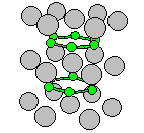Crystal Lattice Structures: Reference Date:  5 Aug 1997 Last Modified: 21 Oct 2004

# The hexagonal ω (C32) StructureYou can now

• This is the hexagonal ω phase. There is also a trigonal ω (C6) phase.
• For more details about the ω phase and materials which form in the ω phase, see S.K. Sikka, Y.K. Vohra, and R. Chidambaram, Progress in Materials Science 27, 245-310 (1982). Most ω phase intermetallic alloys are disordered.
• One interesting thing about this structure is that the B-B distance is smaller than the Al-B distance for every c/a ratio. So if c/a is small enough the structure looks like a set of inter-penetrating Boron triangular planes and Aluminum chains. If c/a = 3-1/3 the Al-Al distance along (001) is the same as the B-B distance in the plane, and, for that matter, the B-B distance in the (001) direction. This value (0.577) is close to the value (3/8)1/2 (0.612) where the trigonal ω phase can transform to the body-centered cubic (A2) lattice, which probably explains the close connection between the ω and bcc phases.
• The other interesting thing about this structure is that the Boron atoms form graphite-like sheets. For this reason, as of 7 Feb 2003, we've moved this structure into the sp2 section of the Carbon and Related Structures page.

• Prototype: AlB2
• Pearson Symbol: hP3
• Strukturbericht Designation: C32
• Space Group: P6/mmm (Cartesian and lattice coordinate listings available)
• Number: 191
• Reference: Villars and Calvert, Pearson's Handbook, Vol. I, p. 656.
• Other systems with this structure: Ti (metastable), MgB2, Be2Hf, CeHg2
• Primitive Vectors:  A1 = ½ a X - ½ 31/2 a Y A2 = ½ a X + ½ 31/2 a Y A3 = c Z
• Basis Vectors:  B1 = 0 (Al) (1a) B2 = 1/3 A1 + 2/3 A2 + ½ A3 = ½ aX + 12-½ a Y + ½ c Z (B) (2d) B3 = 2/3 A1 + 1/3 A2 + ½ A3 = ½ a X - 12-½ a Y + ½ c Z (B) (2d)

### See these vectors as LaTeX output.

Go back to the Carbon and Related Structures page.

Go back to Crystal Lattice Structure page.

 Structures indexed by: This is a mirror of an old page created at theNaval Research LaboratoryCenter for Computational Materials ScienceThe maintained successor is hosted at http://www.aflowlib.org/CrystalDatabase/ and published as M. Mehl et al., Comput. Mater. Sci. 136 (Supp.), S1-S828 (2017).